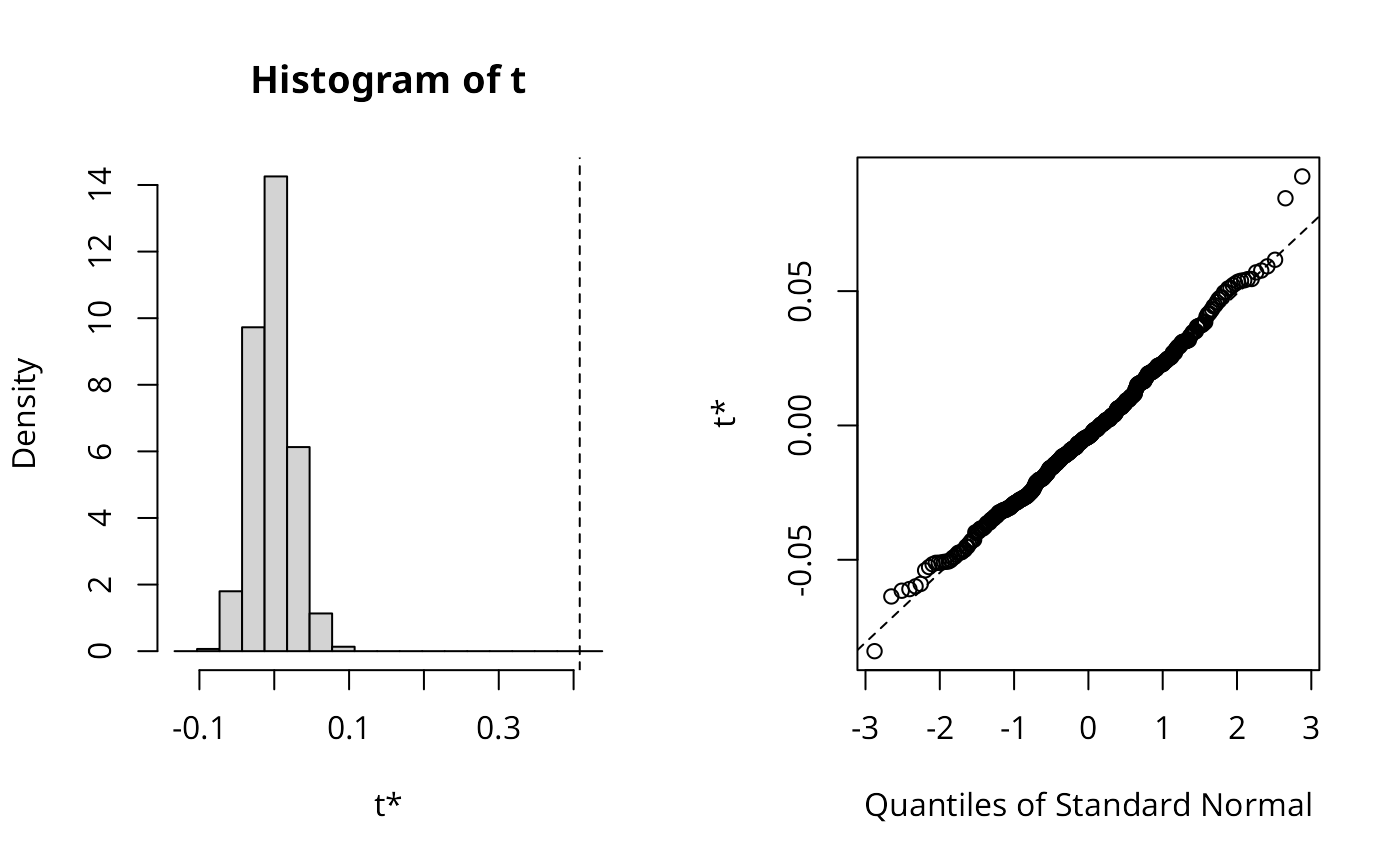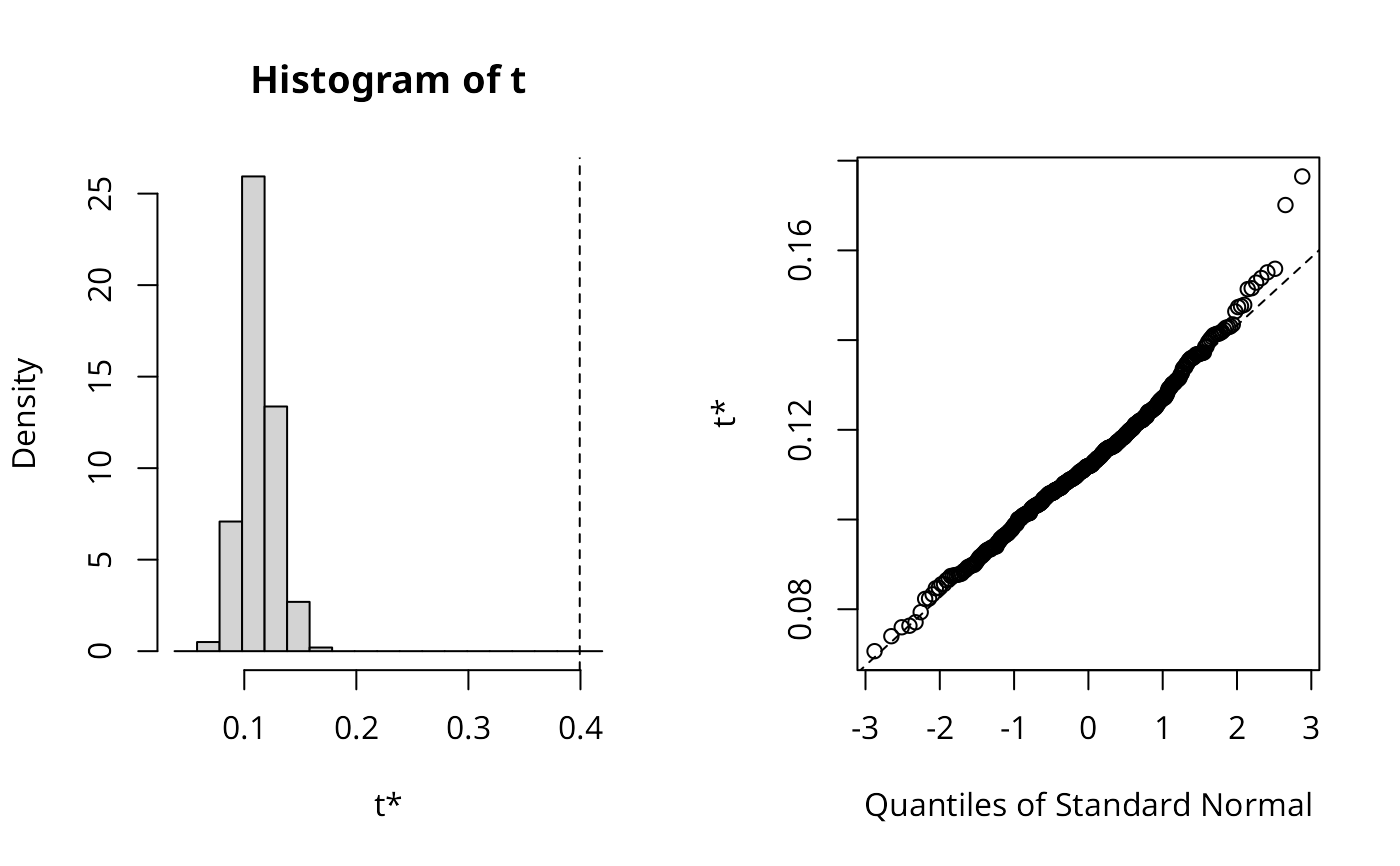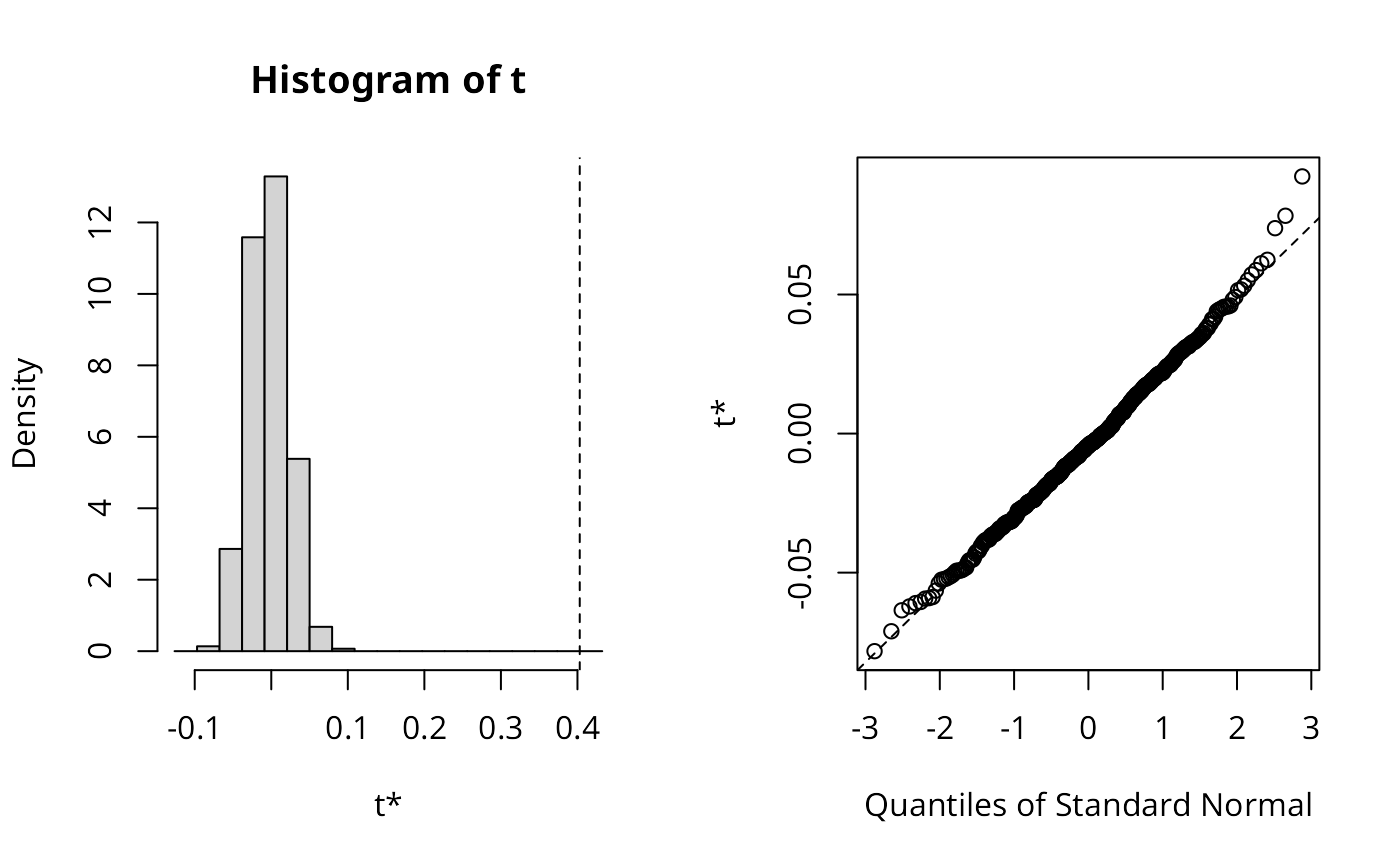Given two continuous numeric variables, calculate the bivariate Moran's I. See details for more.

moran_bv(x, y, listw, nsim = 499, scale = TRUE)

## Arguments

x

a numeric vector of same length as y.

y

a numeric vector of same length as x.

listw

a listw object for example as created by nb2listw().

nsim

the number of simulations to run.

scale

default TRUE.

## Value

An object of class "boot", with the observed statistic in component t0.

## Details

The Global Bivariate Moran is defined as

$$I_B = \frac{\Sigma_i(\Sigma_j{w_{ij}y_j\times x_i})}{\Sigma_i{x_i^2}}$$

It is important to note that this is a measure of autocorrelation of X with the spatial lag of Y. As such, the resultant measure may overestimate the amount of spatial autocorrelation which may be a product of the inherent correlation of X and Y.

## Author

Josiah Parry josiah.parry@gmail.com

## Examples

data(boston, package = "spData")
x <- boston.c$CRIM y <- boston.c$NOX
listw <- nb2listw(boston.soi)
set.seed(1)
res_xy <- moran_bv(x, y, listw, nsim=499)
res_xy$t0 #>  0.4082073 plot(res_xy)set.seed(1) lee_xy <- lee.mc(x, y, listw, nsim=499, return_boot=TRUE) lee_xy$t0
#>  0.3993476
plot(lee_xy)set.seed(1)
res_yx <- moran_bv(y, x, listw, nsim=499)
res_yx$t0 #>  0.4029821 plot(res_yx)set.seed(1) lee_yx <- lee.mc(y, x, listw, nsim=499, return_boot=TRUE) lee_yx$t0
#>  0.3993476
plot(lee_yx)